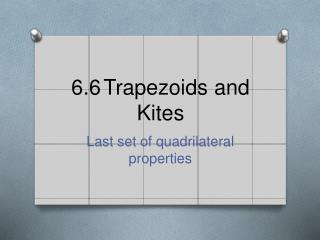# 6.6 Trapezoids and Kites - PowerPoint PPT PresentationDownload Presentation6.6 Trapezoids and Kites

6.6 Trapezoids and KitesDownload Presentation## 6.6 Trapezoids and Kites

- - - - - - - - - - - - - - - - - - - - - - - - - - - E N D - - - - - - - - - - - - - - - - - - - - - - - - - - -
##### Presentation Transcript

1. 6.6 Trapezoids and Kites Last set of quadrilateral properties

2. Terminology:

3. Terminology:

4. Terminology:

5. Terminology:

6. Start with the trapezoid

7. Start with the trapezoid

8. Start with the trapezoid • Parallel sides are called bases

9. Start with the trapezoid • Non parallel sides are called legs.

10. Start with the trapezoid • Since one pair is parallel

11. Start with the trapezoid • Since one pair is parallel Angles on the same leg are supplementary.

12. Now for the special

13. Now for the special • Isosceles trapezoid is a trapezoid whose legs are congruent.

14. And now for the proof, drawing in perpendiculars A B C D E F

15. Why is ? A B C D E F

16. Remember, A B C D E F

17. Why is ? A B C D E F

18. As a result, ACE  BDF by? A B C D E F

19. C  D by… A B C D E F

20. As a result, A  B by… A B C D E F

21. Theorem 6-19: If a quadrilateral is an isosceles trapezoid, then each pair of base ’s is . A B C D E F

22. Make sure you can…

23. Make sure you can… • Given one angle of an isosceles trapezoid, find the remaining 3 angles.

24. Application: page 390 Problem 2

25. Focusing on 1 section

26. AC  BD because? A B E C D

27. C  D by? A B E C D

28. If we want to prove ’s ACD and BCD are congruent, what do they share? A B E C D

29. ACD  BCD by A B E C D

30. AD  BC by A B E C D

31. Theorem 6-20: If a quadrilateral is an isosceles trapezoid, then its diagonals are  A B E C D

32. The return of midsegments  A midsegment of a trapezoid connects the midpoints of the legs (non parallel sides) and is the mean value of the 2 bases (parallel sides)

33. The return of midsegments A midsegment of a trapezoid connects the midpoints of the legs (non parallel sides) and is the mean value of the 2 bases (parallel sides)

34. In addition… A midsegment of a trapezoid connects the midpoints of the legs (non parallel sides) and is the mean value of the 2 bases (parallel sides)

35. In addition… Much like triangles, the midsegment is parallel to the sides it does not touch.

36. So find its length?

37. So find its length? • Add the bases and divide by 2.

38. Working backwards

39. Working backwards • Formula:

40. Working backwards • Formula: • Midsegment =

41. Plug in the length of the midsegment. • Formula: • Midsegment =

42. Plug in the length of a base. • Formula: • Midsegment =

43. Solve for the remaining base • Formula: • Midsegment =

44. Solve for the remaining base • Or • Arithmetically, multiply the length of the midsegment by 2 and subtract the length of the given base.

45. Here’s a problem I enjoy. • Given an isosceles trapezoid whose midsegment measures 50 cm and whose legs measures 24 mm. Find its perimeter.

46. Now to kites:

47. If we drew in a line of symmetry, where would it be?

48. And now are there  ’s?